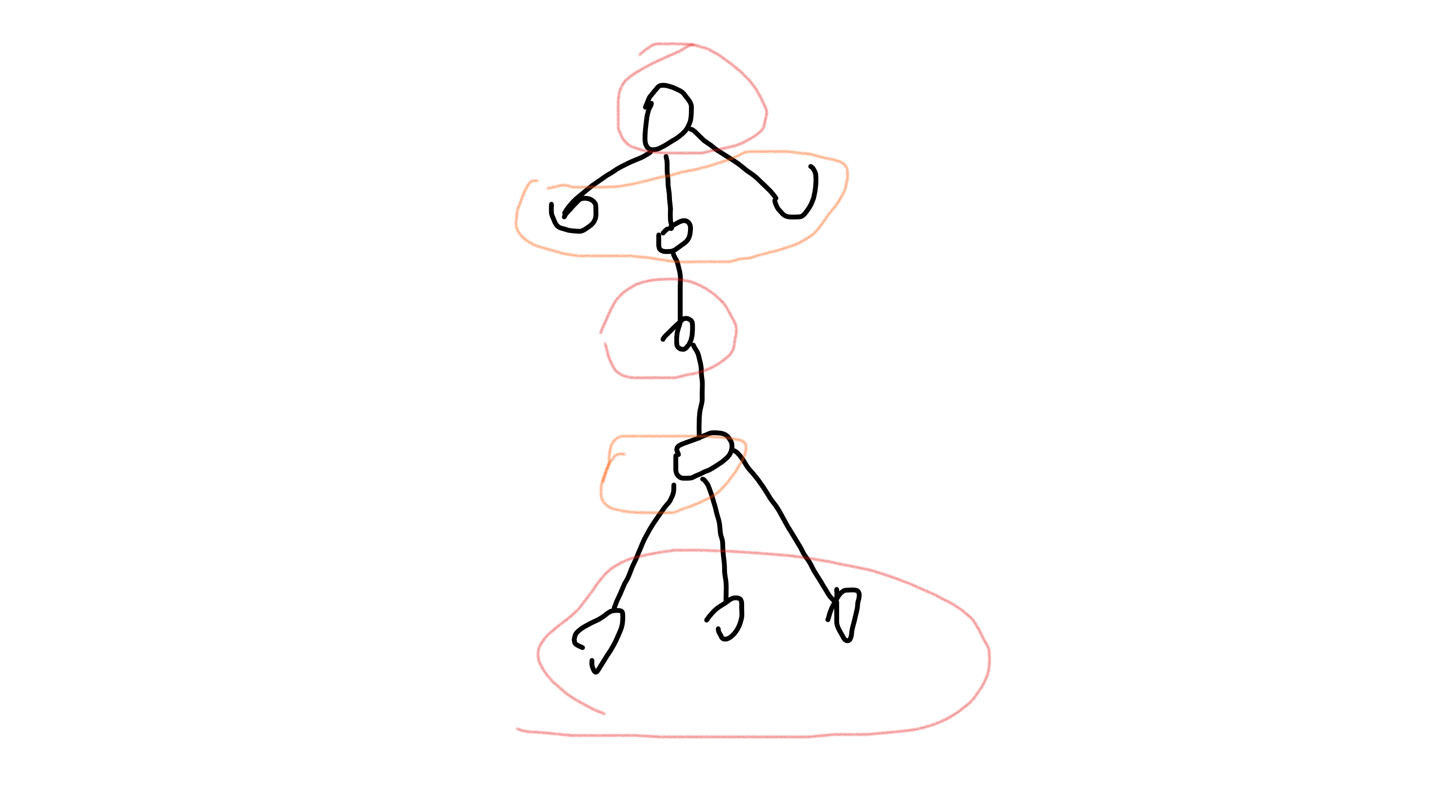# UVA1220 - Party at Hali-Bula

• $f[u] = \sum\limits_{v \in son[u]} \max(f[v], f[v])$
• $f[u] = \sum\limits_{v \in son[u]} f[v]$

• 如果当前节点 $u$ 更新状态选中的来自 $v$ 的某个状态存在多种，那么 $u$ 的这个状态也存在多种。
• 如果 $u$ 节点的某个孩子 $v$ 满足 $f[v] == f[v]$ ，那么 $f[u]$ 存在多种。

# 洛谷2996 - 拜访奶牛• 如果当前节点不选，那么孩子节点选或不选均可
$f[u] += max(f[G[u][i]], f[G[u][i]])$

• 如果当前节点选中，那么孩子节点只能不选
$f[u] += f[G[u][i]]$